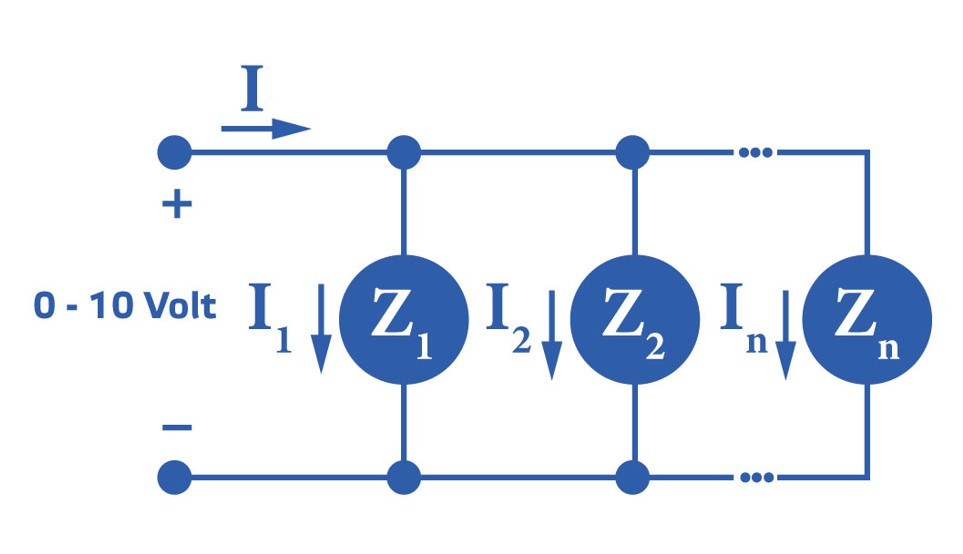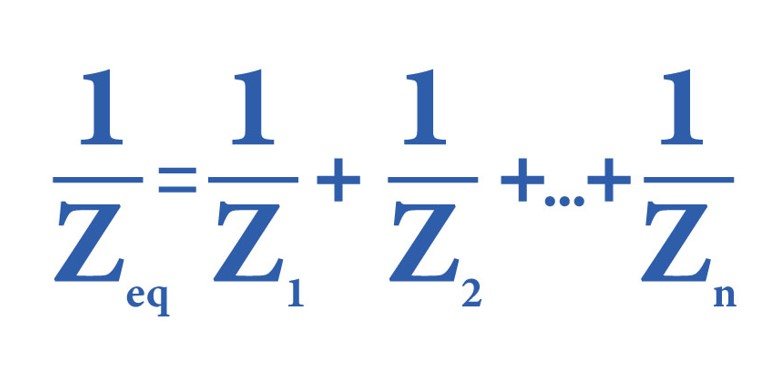Sentera – your partner in HVAC control solutions
Category
• HVAC control solutions
• Customer service
• Senteraweb - Setup, monitoring, logging

# How many EC fans can I control with a 0-10 Volt signal?

Input impedance EC motor
Every power source has a limit, also the source of a 0-10 Volt signal is limited. Sentera expresses the limit of a 0-10 Volt signal as minimum load in the datasheets. E.g.: Analogue output: 0-10 VDC / min. load 1 kΩ When the minimum load is connected to the 0-10 Volt output, the output current will be at its maximum value

This minimum load current depends on the input impedance of the connected EC motor(s). This value is mentioned in the technical specifications of the EC fan or can be requested from the EC fan manufacturer. The input impedance is expressed in Ohms or in Kilo Ohms. 1 Kilo Ohm = 1000 Ohm.To calculate the maximum current, apply Ohm's law: U [Volt] = R [Ohm] x I [Amperes]

Let's just assume that impedance (Z) and resistance (R) are identical to simplify things. This means that:  I = U / Z
If the EC fan has an input impedance of 1.000 Ω this becomes: 10 VDC / 1 kΩ = 0,01 A = 10 mA.

When the control signal is 10 Volt and the EC fan has an input impedance of 1000 Ω, a current of 10 mA will run through the wires of the 0-10 Volt signal.

How to calculate the combined impedance of multiple EC motors?
If you want to regulate multiple EC motors with a 0-10 Volt signal, you must connect the 0-10 Volt signal in parallel to the EC motors. Each 0-10 Volt input of the connected EC motors has a certain input impedance (Z1, Z2, etc.). To calculate the maximum number of EC motors that can be controlled by the 0-10 Volt signal, we must calculate the total input impedance Zeq of all connected EC motors. The total or equivalent input impedance (Zeq [Ω]) can be calculated using this formula:An example: When 3 identical EC motors - each with an input impedance of 600 Ω - are connected in parallel, their combined equivalent impedance is: 1/Zeq = 1/600 Ω + 1/600 Ω +1/600 Ω = 3/600 Ω
Thus, their combined impedance Zeq is 200 Ω.
When the minimum load of the 0-10 Volt signal is 200 Ω and cable lengths remain limited, it will be possible to control the 3 EC motors simultaneously via this 0-10 Volt signal.